Module 17 - Applications of Integration Introduction | Lesson 1 | Lesson 2 | Lesson 3 | Self-Test Lesson 17.2: Total Area and The Area Between Two Curves This lesson explores finding the total area under a curve and the area between two curves. The total area of a region that is both above and below the x-axis is found by separating the positive parts of the graph from the negative parts. Each part is integrated and the absolute values of the results are added together to find the total area. Examine the graph of y = sin x from 0 to 2again.As shown in Lesson 17.1,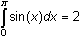, and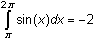. The total area bounded by y = sin x on the interval [0, 2] is 2 + | -2 | = 4 square units. Using Absolute Value to Find Total Area Another method used to find the total area is to integrate the absolute value of the function. You can find the absolute value, abs(), under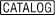17.2.1 Evaluate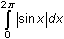and interpret the result. Click here for the answer. 17.2.2 Find the total area bounded by y = x3 – 3x2 – x + 3 and the x-axis on the interval [0, 4]. Click here for the answer. Finding the Area between Two Curves Integrals can be used to find the area between two curves by evaluating the integral of the difference between the upper curve and the lower curve between their points of intersection. Find the area between y = 4 – x2 and y = x by following the procedure below. Graph the two functions y = 4 – x2 and y = x in a [-3, 3] x [-5, 5] window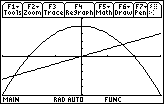The upper curve is y = 4 – x2 and the lower curve is y = x. Find the points where the curves intersect. Solve(4-x^2=x,x)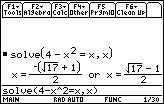The area between the curves is given by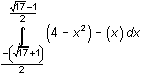17.2.3 Evaluate the integral above on your TI-89 and interpret the result. Click here for the answer. < Back | Next > ©Copyright 2007 All rights reserved. | Trademarks | Privacy Policy | Link Policy